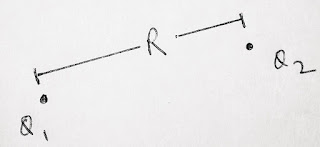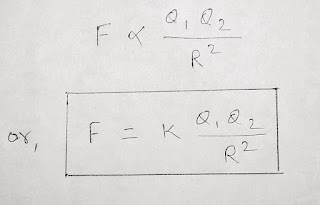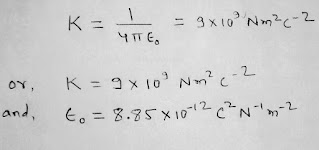# ELECTRIC CHARGE & INVERSE SQUARE LAW:

### Electric charge :

Electric charge is a basic property of matter which gives rise to electric force.

Just like mass of a body responsible for  gravitational force similarly electric charges are responsible for electrostatic or electric force.

• there are two kinds of charges positive and negative.

• Charges are quantized in nature.

• Similar charges repel each other while opposite charges attract each other.

•  the minimum charge a particle can acquire is 1.6 ×10-19 coulombs.

• coulomb ( C ) is SI unit of charge.

### Coulomb's inverse square law :

It states that the force of interaction between two charges depends directly on the product of two charges and  inversely on the square of the distance between them.if Q1 and Q2 are two point charges separated by a distance R then according to coulomb's law the force of interaction between them is

F  α  Q1×Q2 ……………….          (1)

&.    F  α   1/R2 ………………..          ( 2 )

Combining equation 1 & 2 we get,here k is constant of proportionality and is given byand ε₀ = Absolute permittivity of free space

Using above value of k we get,……..(3)

Equation 3 is the required equation for coulomb's inverse square law.

### Unit Charge :

We can define unit charge by using equation 3.

We suppose Q1 =Q2 =Q = 1 C;

And R = 1 m ;

In that case from equation 3 we simply get

F= 9 × 10 9 N ( since k = 9 × 10 9 Nm2C-2 ).

Hence, Unit charge (in SI i.e. 1 coulomb) is that much charge which when placed in vacuum at a distance of 1 m from an equal and similar charge would repel it with a force of 9 × 10 9 N .

1.